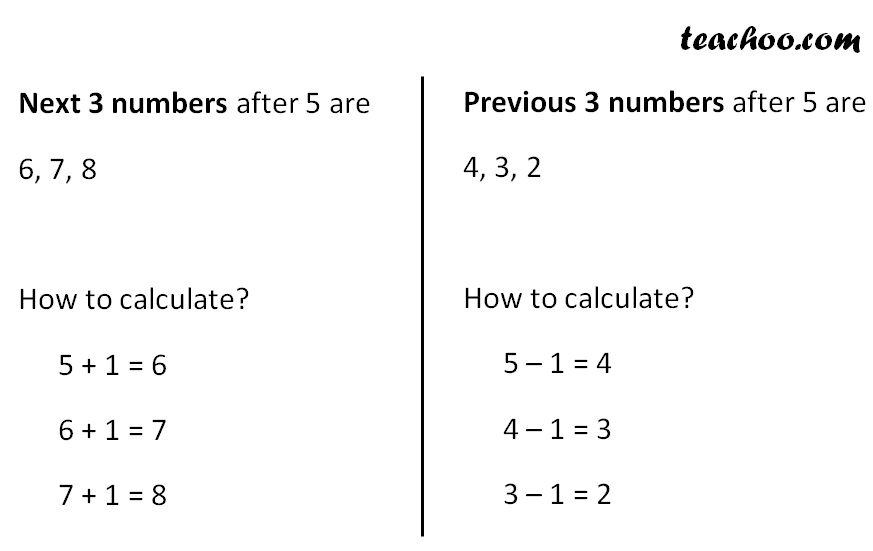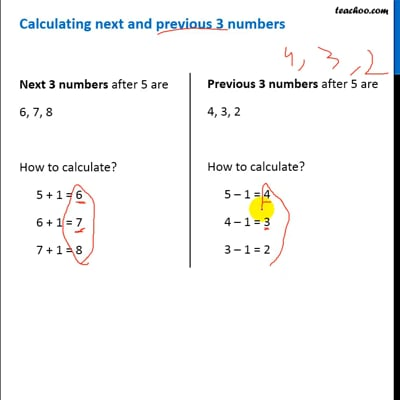Definition

Chapter 2 Class 6 Whole Numbers
Concept wise

## Calculating next and previous 3 numbersThis video is only available for Teachoo black users

Get live Maths 1-on-1 Classs - Class 6 to 12

### Transcript

Calculating next and previous 3 numbers Next 3 numbers after 5 are 6, 7, 8 How to calculate? 5 + 1 = 6 6 + 1 = 7 7 + 1 = 8 Previous 3 numbers after 5 are 4, 3, 2 How to calculate? 5 – 1 = 4 4 – 1 = 3 3 – 1 = 2